# Let A be a diagonalizable n x n matrix and let P be an invertible n...Let A be a diagonalizable n x n matrix and let P be an invertible n x n matrix such that B = p-1AP is the diagonal form of A. Prove that A* = Pokp-1, where k is a positive integer. Use the result above to find the indicated power of A. 10 18 A = -6 -11 18].46 A = 11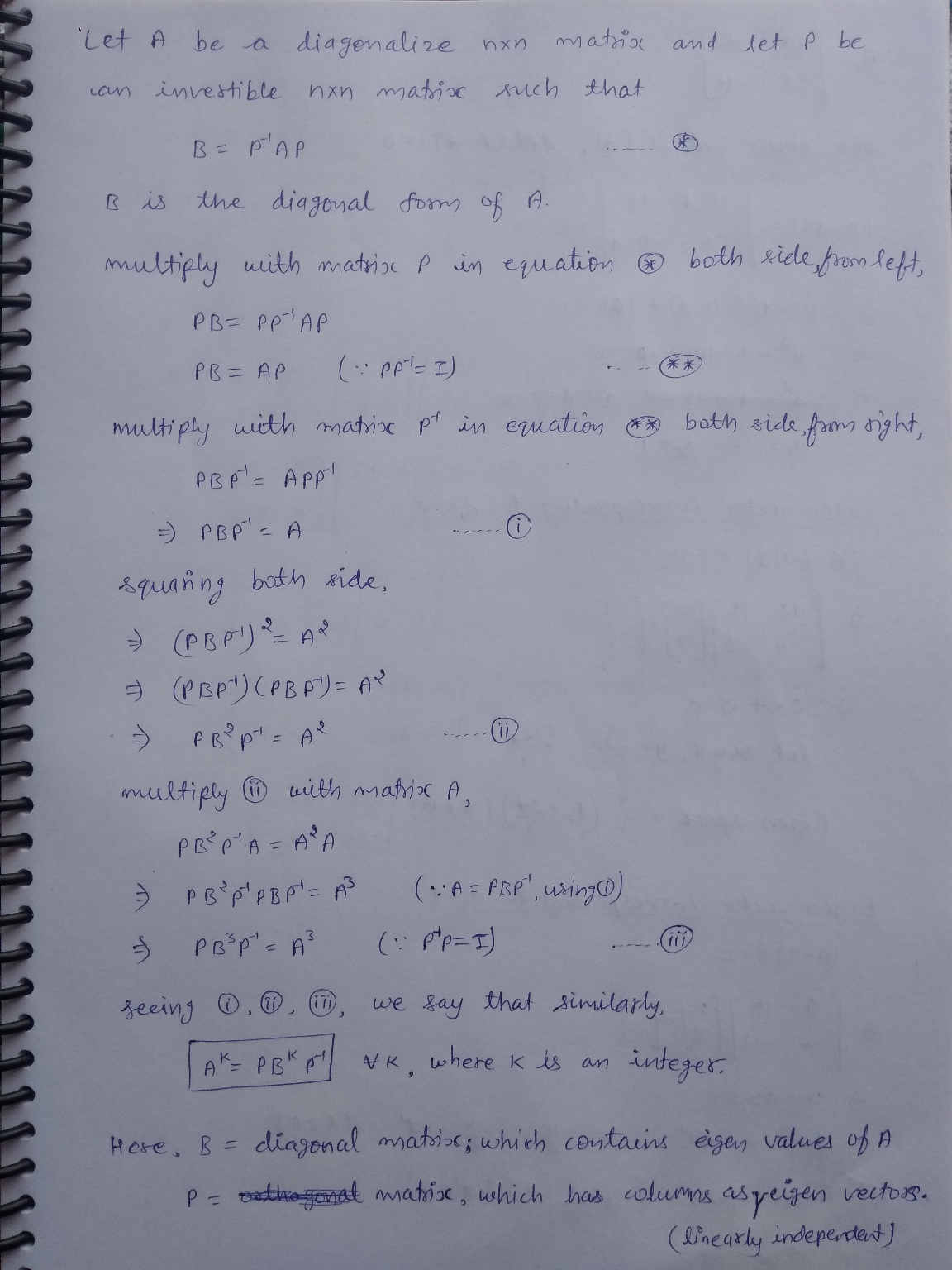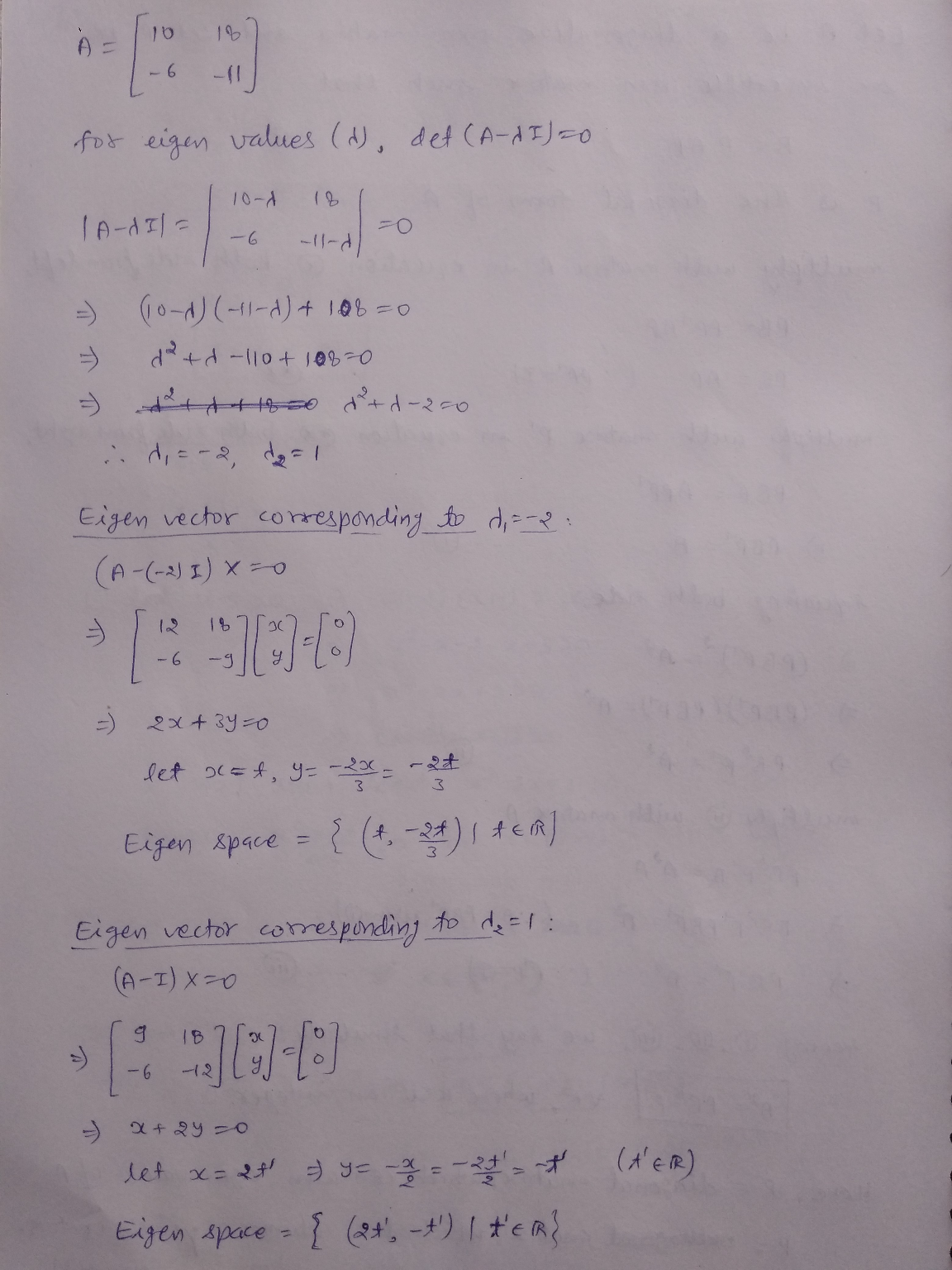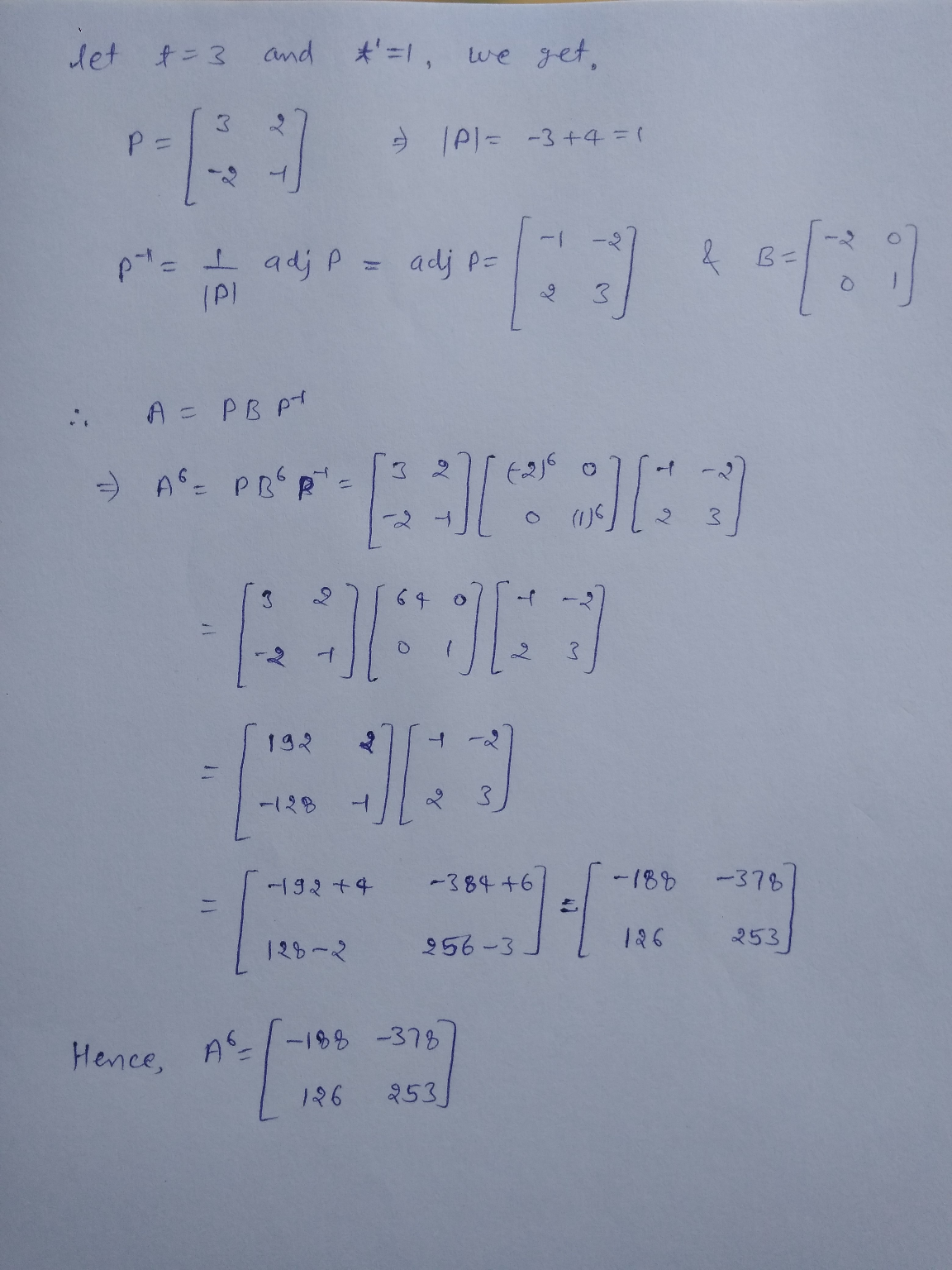#### Earn Coin

Coins can be redeemed for fabulous gifts.

Similar Homework Help Questions
• ### Let A be a diagonalizable n x n matrix and let P be an invertible n...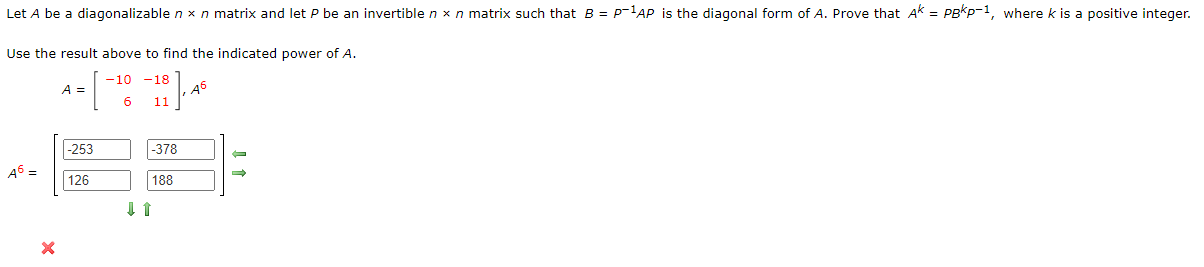Let A be a diagonalizable n x n matrix and let P be an invertible n x n matrix such that B = p-1AP is the diagonal form of A. Prove that Ak = pokp-1, where k is a positive integer. Use the result above to find the indicated power of A. -10 -18 A = 6 11 18].45 -253 -378 A6 = 126 188 11

• ### Let A be a diagonalizable n x n matrix and let P be an invertible n...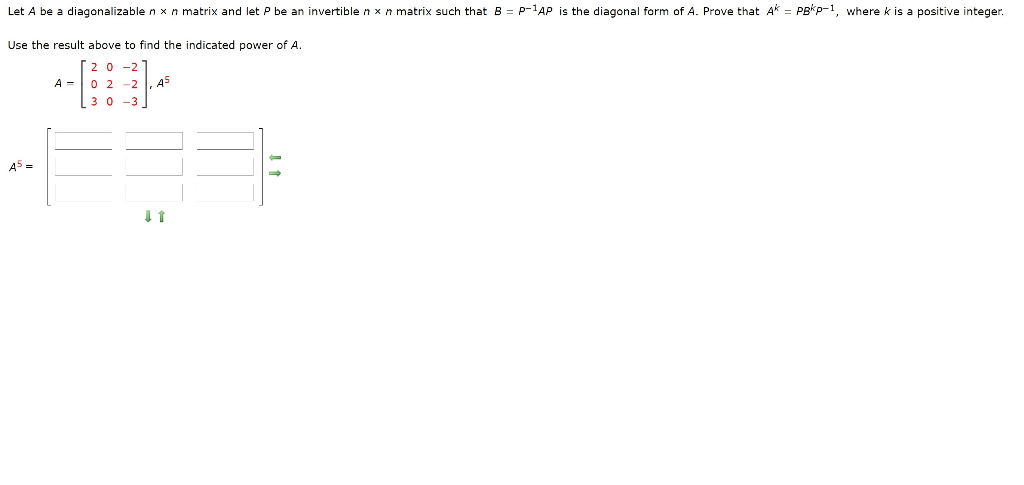Let A be a diagonalizable n x n matrix and let P be an invertible n x n matrix such that B = P-1AP is the diagonal form of A. Prove that Ak = Pekp-1, where k is a positive integer. Use the result above to find the indicated power of A. 0-2 02-2 3 0 -3 ,45 A5 = 11

• ### Let A be a diagonalizable n × n matrix and let P be an invertible n...

Let A be a diagonalizable n × n matrix and let P be an invertible n × n matrix such that B = P−1AP is the diagonal form of A. Prove that Ak = PBkP−1, where k is a positive integer. Use the result above to find the indicated power of A. A = −4 0 4 −3 −1 4 −6 0 6 , A5

• ### Q19 (18) Let A--7 10 1 Find a matrix P, scalar r, and angle α such that P-1AP-r -4 5 -sin α cos α cos α sin α (19) Prove: If A is an invertible n x n-matrix and λ is an eigenvalue of A, then...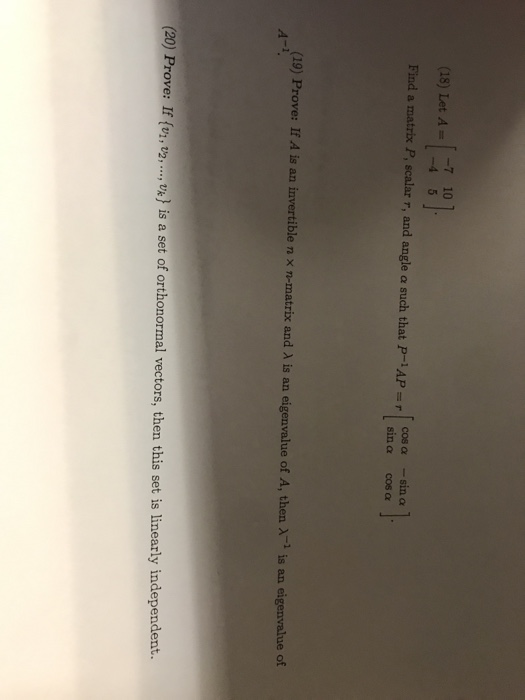Q19 (18) Let A--7 10 1 Find a matrix P, scalar r, and angle α such that P-1AP-r -4 5 -sin α cos α cos α sin α (19) Prove: If A is an invertible n x n-matrix and λ is an eigenvalue of A, then λ-1 is an eigenvalue of (20) Prove: If (vi, t2,.,, 'x) is a set of orthonormal vectors, then this set is linearly independent. г_7 10 (18) Let A 4 5 Find a matrix P,...

• ### Suppose that A is diagonalizable and all eigenvalues of A are positive real numbers. Prove that...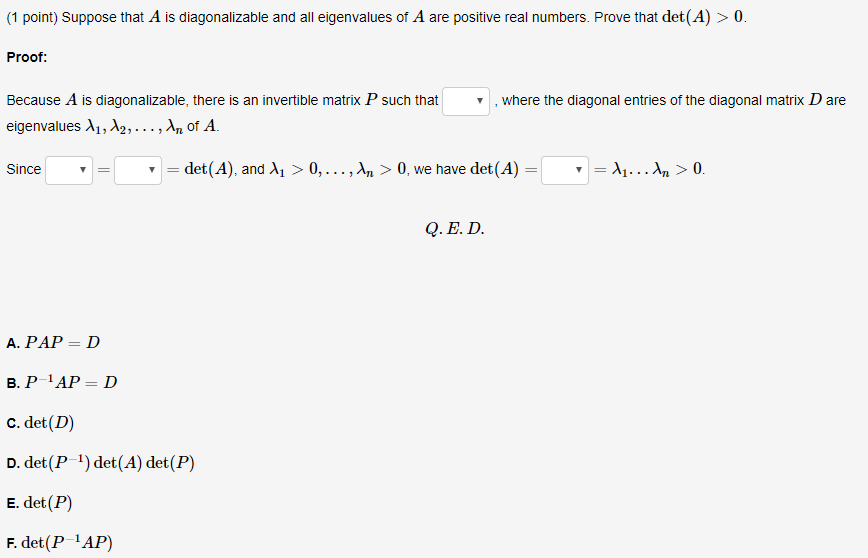Suppose that A is diagonalizable and all eigenvalues of A are positive real numbers. Prove that det (A) > 0. (1 point) Suppose that A is diagonalizable and all eigenvalues of A are positive real numbers. Prove that det(A) > 0. Proof: , where the diagonal entries of the diagonal matrix D are Because A is diagonalizable, there is an invertible matrix P such that eigenvalues 11, 12,...,n of A. Since = det(A), and 11 > 0,..., n > 0,...

• ### Diagonalize the matrix A. That is, find an invertible matrix P and a diagonal matrix D such that

Let A be the matrix4 -12 1Diagonalize the matrix A. That is, find an invertible matrix P and a diagonal matrix Dsuch thatP^-1AP = DFind P^-1.... Use the factorization A = PDP^-1 to compute A^5please explain

• ### Let A = CD where C, D are n xn matrices, and is invertible. Prove that...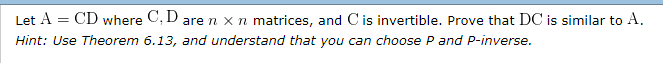Let A = CD where C, D are n xn matrices, and is invertible. Prove that DC is similar to A. Hint: Use Theorem 6.13, and understand that you can choose P and P-inverse. Prove that if A is diagonalizable with n real eigenvalues 11, 12,..., An, then det(A) = 11. Ay n Prove that if A is an orthogonal matrix, then so are A and A'.

• ### 37 40 -120 1 point) Let 5 -815Find an invertible matrix P and a diagonal matrix...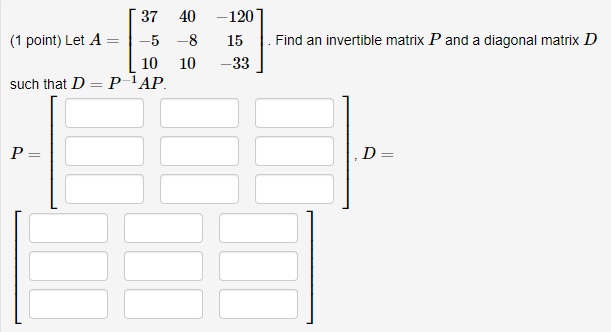37 40 -120 1 point) Let 5 -815Find an invertible matrix P and a diagonal matrix D 10 10 -33 such that D P-1AP

• ### Problem 1. Let A be an m x m matrix. (a) Prove by induction that if A is invertible, then for every n N, An is invertible. (b) Prove that if there exists n N such that An is invertible, then A is inv...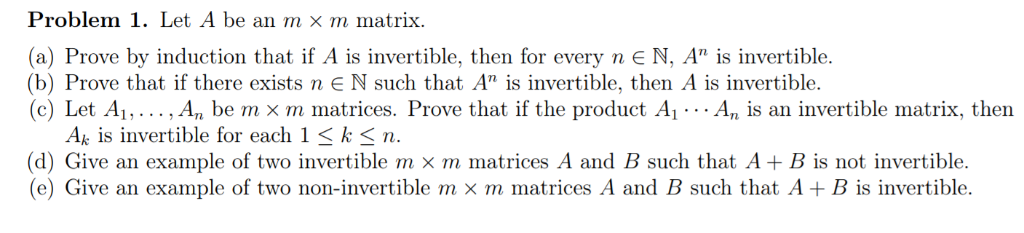Problem 1. Let A be an m x m matrix. (a) Prove by induction that if A is invertible, then for every n N, An is invertible. (b) Prove that if there exists n N such that An is invertible, then A is invertible. (c) Let Ai, . . . , An be m x m matrices. Prove that if the product Ai … An is an invertible matrix, then Ak is invertible for each 1 < k< n. (d)...

• ### please solve both 3. [-12 Points] DETAILS LARLINALG8 7.2.007. For the matrix A, find (if possible)...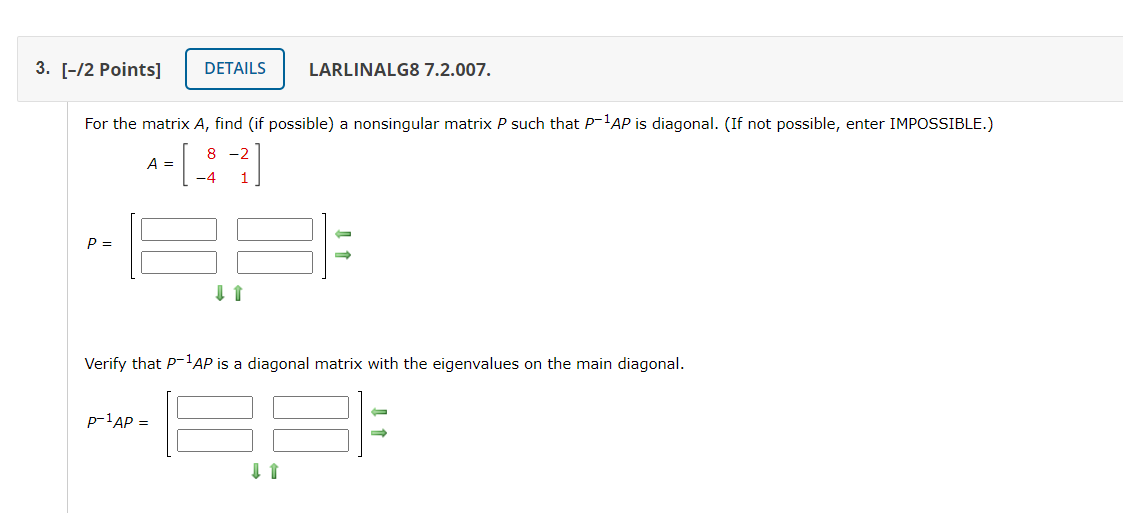please solve both 3. [-12 Points] DETAILS LARLINALG8 7.2.007. For the matrix A, find (if possible) a nonsingular matrix P such that p-1AP is diagonal. (If not possible, enter IMPOSSIBLE.) 8 -2 A= P= Verify that P-1AP is a diagonal matrix with the eigenvalues on the main diagonal. p-1AP = 1. [0/2 Points] DETAILS PREVIOUS ANSWERS LARLINALG8 7.2.001. Consider the following. -11 40 A= -27 (a) Verify that A is diagonalizable by computing p-1AP. -1 0 p-1AP = 10 3...

Free Homework App